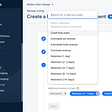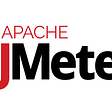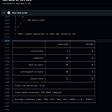# Orion: A next-generation automation testing tool

Orion is born to help us to write and automate our acceptance tests. It provides a DSL inspired by Gherkin and based in HCL.

The goal of Orion, is to provide people without technical skills a tool to write acceptance tests.

In this article, we will go through some real scenarios to learn how to deal with Orion.

# Setup Orion

To follow the article I highly recommend you to get Orion installed on your computer. It will take you less than a minute

# Showcase I: math operations

We will write acceptance tests to verify that operation `add`and `subtract`work correctly.

## Initial approach

We will write a couple of scenarios to verify the below:

• 10 + 5 = 15
• 10 -5 = 5
`# feature-math-operations.hcldescription = <<EOF   This feature is used to demonstrate that both add and subs   operations work as expected.EOFscenario "operation add" {   given "the variables x and y" {      set x {         value = 10      }      set y{         value = 5      }   }   when "values are added" {      set result {         value = x + y      }      print {         msg = "\${x} + \${y} is \${result}"      }   }   then "the result of the operation is the expected" {      assert {         assertion = result==15      }   }}scenario "operation substract" {   given "variables x and y" {      set x {         value = 10      }      set y{         value = 5      }   }   when "subtract y to x" {      set result {         value = x - y      }      print {         msg = "\${x} - \${y} is \${result}"     }   }   then "the result of the operation is the expected" {      assert {         assertion = result==5      }   }}`

Let’s execute`orion run --input feature-math-operations.hcl`

## Hooks

Hooks allow us to write a reusable set of actions that can be executed before or after the scenarios. Visit the Hooks documentation

We’ll use the special hook after each to print the operation result. As a consequence, we can remove the action `print`in the section when from the scenarios. The hook will be executed after any scenario is completed.

`# feature-math-operations.hcldescription = <<EOF   This feature is used to demonstrate that both add and subs   operations work as expected.EOFafter each {   print {      msg = "the output of this operation is \${result}"   }}scenario "operation add" {   given "the variables x and y" {      set x {         value = 10      }      set y{         value = 5      }   }   when "values are added" {      set result {         value = x + y      }   }   then "the result of the operation is the expected" {      assert {         assertion = result==15      }   }}scenario "operation substract" {   given "variables x and y" {      set x {         value = 10      }      set y{         value = 5      }   }   when "subtract y to x" {      set result {         value = x - y      }   }   then "the result of the operation is the expected" {      assert {         assertion = result==5      }   }}`

Let’s execute`orion run --input feature-math-operations.hcl`

## Input variables

A better approach is to execute the same scenarios with different data. We can do this with block `input.` Visit the Input arguments Documentation

`# feature-math-operations.hcldescription = <<EOF   This feature is used to demonstrate that both add and subs   operations work as expected.EOFinput {   arg x {      default = 10   }   arg y {      default = 5       }   arg sumResult {      default = 15       }   arg subResult {      default = 5       }}after each {   print {      msg = "the output of this operation is \${result}"   }}scenario "operation add" {   when "values are added" {      set result {         value = x + y      }   }   then "the result of the operation is the expected" {      assert {         assertion = result==sumResult      }   }}scenario "operation substract" {   when "subtract y to x" {      set result {         value = x - y      }   }   then "the result of the operation is the expected" {      assert {         assertion = result==subResult      }   }}`

Since we defined default values for all the args we could just run the features as usual:`orion run --input feature-math-operations.hcl`

On the other hand, we can set values for variables, as’s shown below

`# variables-math-operations.hclx = 54y = 46sumResult = 100subResult = 8`

Let’s execute`orion run --input feature-math-operations.hcl --vars vars-math-operations.hcl`

## Skip scenarios

Attribute `ignore` permits us to skip the execution of a scenario. For example, we can establish that the second scenario is ignored when `x>10.`

`# feature-math-operations.hcldescription = <<EOF   This feature is used to demonstrate that both add and subs   operations work as expected.EOFinput {   arg x {      default = 10   }   arg y {      default = 5       }   arg sumResult {      default = 15       }   arg subResult {      default = 5       }}after each {   print {      msg = "the output of this operation is \${result}"   }}scenario "operation add" {   when "values are added" {      set result {         value = x + y      }   }   then "the result of the operation is the expected" {      assert {         assertion = result==sumResult      }   }}scenario "operation substract" {   ignore = x > 10   when "subtract y to x" {      set result {         value = x - y      }   }   then "the result of the operation is the expected" {      assert {         assertion = result==subResult      }   }}`

If we don’t pass the variables file both scenarios will be executed because the default value of x is 10. On the other hand, if we pass the variables file, the second scenario won’t be executed because x is 54.

## Includes

We can split the content of our file into several files, and reuse them for different features. Visit the Includes Documentation. To put into practice this, we will move the scenarios to separated files.

`# scenario-sum.hclscenario "operation add" {   when "values are added" {      set result {         value = x + y      }   }   then "the result of the operation is the expected" {      assert {         assertion = result==sumResult      }   }}# scenario-sub.hclscenario "operation substract" {   ignore = x > 10   when "subtract y to x" {      set result {         value = x - y      }   }   then "the result of the operation is the expected" {      assert {         assertion = result==subResult      }   }}`

and then, we just make use of attribute `includes`

`# feature-math-operations.hcldescription = <<EOF   This feature is used to demonstrate that both add and subs   operations work as expected.EOFinput {   arg x {      default = 10   }   arg y {      default = 5       }   arg sumResult {      default = 15       }   arg subResult {      default = 5       }}after each {   print {      msg = "the output of this operation is \${result}"   }}includes = [   "scenario-sum.hcl",    "scenario-sub.hcl"]`

Let’s execute`orion run --input feature-math-operations.hcl`

## Conditional actions

The attribute `when` allows us to define if the action is executed or not. All the actions have this optional attribute. We make the following change in the second scenario: x-y when x>y, and y-x otherwise

`# scenario-sub.hclscenario "operation substract" {   when "subtract y to x" {      set result {         value = x - y         when = x > y           }      set result {         value = y - x         when = x <= y           }   }   then "the result of the operation is the expected" {      assert {         assertion = result==subResult      }   }}`

if we swap the values of x and y in file vars-math-operations.hcl

`# variables-math-operations.hclx = 46 y = 54sumResult = 100subResult = 8`

the execution of our scenarios will be successful

Let’s execute`orion run --input feature-math-operations.hcl --vars vars-math-operations.hcl`

## Multiple cases

If you’re experienced working with Cucumber, this will remember to the scenario outline. We just need to define a set of input data in attribute `examples` in the scenario. For example, we will provide examples for the second scenario.

`# scenario-sub.hclscenario "operation substract" {      examples = [      { x = 20, y = 10, subResult= 10},      { x = 10, y = 20, subResult= 10},      { x = 5, y = 5, subResult= 0},   ]   when "subtract y to x" {      set result {         value = x - y         when = x > y           }      set result {         value = y - x         when = x <= y           }   }   then "the result of the operation is the expected" {      assert {         assertion = result==subResult      }   }}`

if we execute Orion, the above scenario will be executed three times.

Let’s execute`orion run --input feature-math-operations.hcl`

## Special action: block

Let’s implement a new scenario to demonstrate how multiplication works. Initially, we could go for something like this

`# scenario-mult.hclscenario "operation multiplication" {    examples = [      { x = 20, y = 10, multResult= 10},      { x = -1, y = -2, multResult= 2},      { x = 5, y = 5, multResult= 25},      { x = 5, y = 0, multResult= 0},      ]   when "multiply y by x" {      set result {         value = x * y      }   }   then "the result of the operation is the expected" {      assert {         assertion = result==multResult      }   }}`

but I want to show you how the special action block (Documentation here) works. This action is used to group a set of actions. And additionally, we will make use of an attribute `count` that permits iterate from 0 to count-1.

`# scenario-mult.hclscenario "operation multiplication" {    examples = [      { x = 20, y = 10, multResult= 200},      { x = -1, y = -2, multResult= 2},      { x = 5, y = 5, multResult= 25},      { x = 5, y = 0, multResult= 0},      ]      given "initialie result" {      set result {         value = 0      }   }   when "multiply y by x" {      block {         set result {            value = result + x         }         print {            msg = "\${x} * \${_.index+1} is \${result}"         }         count = y         when = x>0 && y>0           }      set result {        value = x * y             when = x<0 || y<0     }   }   then "the result of the operation is the expected" {      assert {         assertion = result==multResult      }   }}`

And of course, we need to add this file in the include block.

`# feature-math-operations.hcldescription = <<EOF   This feature is used to demonstrate that both add and subs   operations work as expected.EOFinput {   arg x {      default = 10   }   arg y {      default = 5       }   arg sumResult {      default = 15       }   arg subResult {      default = 5       }}after each {   print {      msg = "the output of this operation is \${result}"   }}includes = [   "scenario-sum.hcl",    "scenario-sub.hcl",   "scenario-mult.hcl"]`

Let’s execute`orion run --input feature-math-operations.hcl`

Beside of `when` and `count` there are other special attributes. See the full list here.

Orion is still in beta, hence your suggestions and feedback will be very appreciated!

--

--

--

## More from Iván Corrales Solera

If tech can change the world... why don't we use it to make it better? https://www.linkedin.com/in/ivan-corrales-solera/

Love podcasts or audiobooks? Learn on the go with our new app.

## Why Java has been “dying” for 25 years but is still here## Data Structures Written In Python and their respective LeetCode questions## Top 9 Websites To Find High-Quality Remote Freelance PHP Jobs## EventChain## How I earned \$\$\$\$ by Amazon S3 Bucket misconfigurations?## The 10 Limitations of Firebase A/B Testing (ft. Hackle A/B Testing)## Iván Corrales Solera

If tech can change the world... why don't we use it to make it better? https://www.linkedin.com/in/ivan-corrales-solera/

## Run JMeter Test on a Linux VM on Azure: Step-by-step guide with Linux commands- Part 1(Setup)## Dogfooding Testkube — Part1 — How to Test a Testing Framework — Kubeshop## Continuous Testing Using Shift Left Testing Approach